• Implementing search is always challenging but not impossible.

In real-life, we will search in no pattern. We just go to the places where we think it might be placed. We don’t follow any pattern in most cases.

Does the same thing work in the programming world?

No! there has to some pattern to search things in programs. We are going to see some algorithms which follow different patterns for searching in this article.

There are multiple algorithms that we can find in the programming world. We are going to discuss the most important and mostly used algorithms in this article. And rest of the algorithms will be a piece of cake for you to learn.

Searching refers to searching an element in the array in this article.

Let’s see them one by one.

## Linear Search

The name suggests that the linear search algorithm follows the linear pattern to search the elements in an array. The algorithm starts searching the element from the beginning of the array and moves to the end until it finds the element. It stops the execution of the program when it finds the required element.

Let’s illustrate the linear search algorithms with some cool illustrations for a better understanding of the algorithm.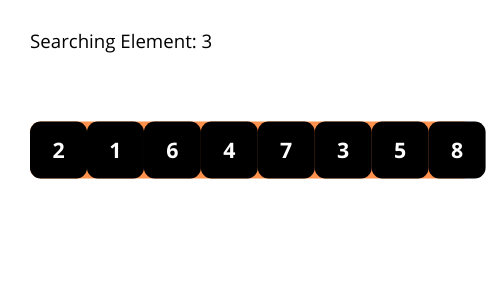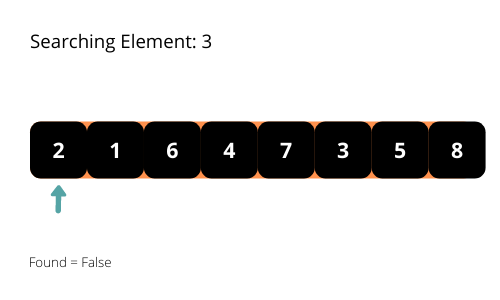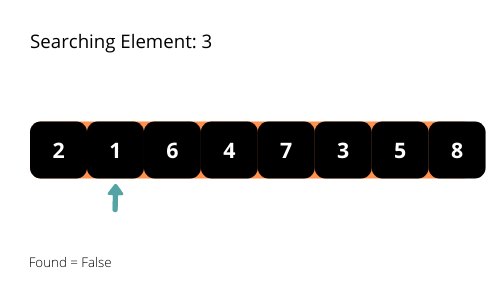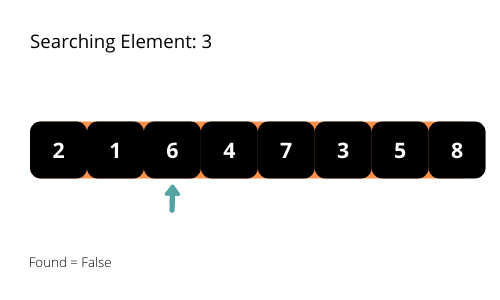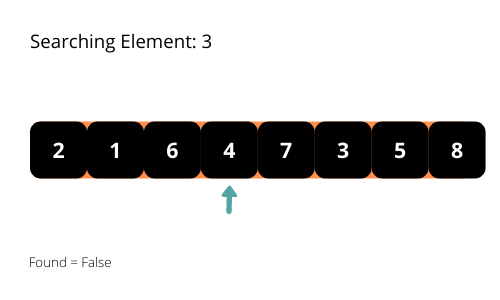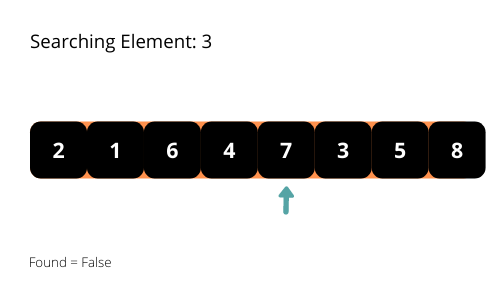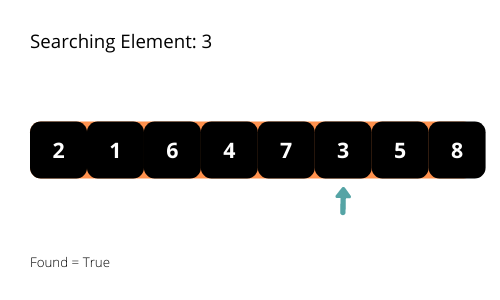If you carefully observe the search pattern, you will find that the time takes for the program’s execution will take O(n) in the worst-case. We have to consider the worst-case time complexity of the algorithms to analyze. Hence the time complexity of the algorithm is O(n).

Let’s see the implementation of the linear search algorithm.

### Linear Search Implementation

Now, you have a good understanding of the linear search algorithm. It’s time to make our hands dirty with some code. Let’s see the steps to implement the linear search first. Then you try to code it. Don’t worry about even if you can’t; I will provide you with the code afterward.

Let’s see the steps to implement the linear search algorithm.

• Initialize an array of elements (your lucky numbers).
• Write a function called search_element, which accepts three arguments, array, length of the array, and element to be searched.
• search_element(arr, n, element):
• Iterate over the given array.
• Check whether the current element equal to the required element.
• If yes, then return True.
• After completing the loop, if the execution is still in the function, then the element is not present in the array. Hence return False.
• Print the message based on the return value of the function search_element.

Finally, write the code with the help of the above steps to implement the linear search algorithm.

Did you complete the implementation of the linear search algorithm? I hope so. If you completed, then cross-check with the following code.

If you didn’t complete it, don’t worry,; see the below code and learn how we implemented it. You will get it without much effort.

``````## searching function
def search_element(arr, n, element):

## iterating through the array
for i in range(n):

## checking the current element with required element
if arr[i] == element:
## returning True on match
return True

return False

if __name__ == '__main__':
## initializing the array, length, and element to be searched
arr = [1, 2, 3, 4, 5, 6, 7, 8, 9, 10]
n = 10
element_to_be_searched = 6
# element_to_be_searched = 11

if search_element(arr, n, element_to_be_searched):
print(element_to_be_searched, "is found")
else:
``````

First, execute the program with an element that is present in the array. And next, execute it with an element that is not present in the array.

The time complexity of the linear search algorithm is O(n).

Can we reduce it a bit further with different patterns?

Yeah, we can. Let’s see it.

## Binary Search

The binary search algorithm always checks the middle element of the array. This algorithm searches the element in a sorted array.

The binary search algorithm iterates over the array and checks the middle element, if found, then stops the program. Else if the middle element is less than the required element, then it omits the left part of the array from the middle element from searching. Else if the middle element is greater than the required element, then it omits the right part of the array from the middle element from searching.

In each iteration, the binary search algorithm cuts down the area to search the element. So, the number of checks is less than the number of checks made in the linear search algorithm.

Illustrations help us in better understanding the binary search algorithm. Let’s check them.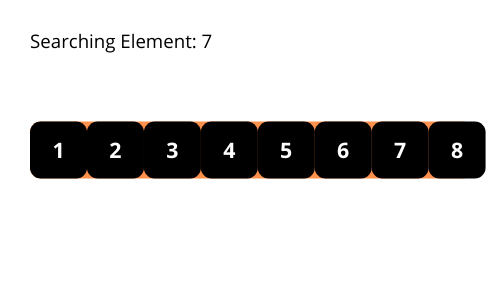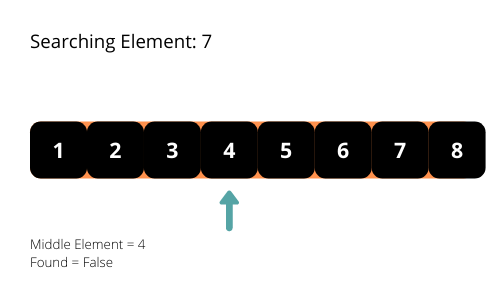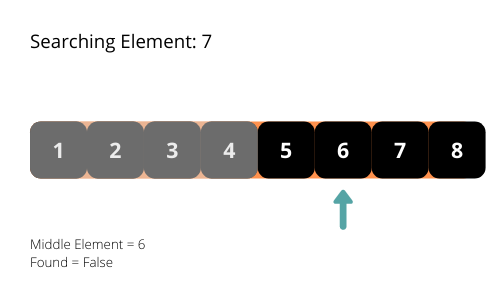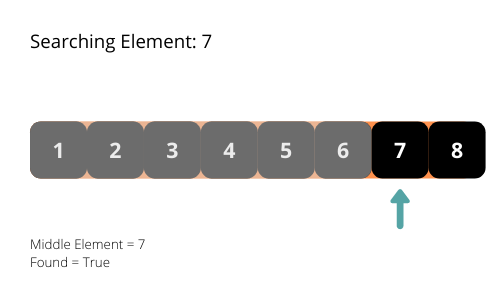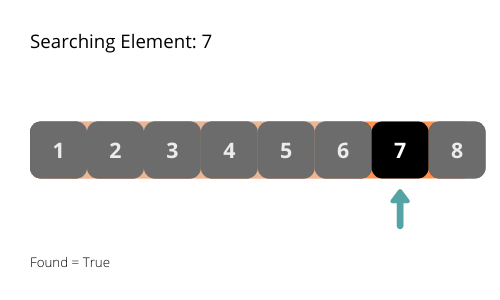The time complexity of the binary search algorithm is O(log n). In each iteration, the length of the search area is reducing by half. It is reducing exponentially.

### Binary Search Implementation

First, we will see the steps to implement the binary search algorithm and then the code. Let’s see the steps to complete the binary search algorithm implementation.

• Initialize the array with elements (your lucky numbers)
• Write a function called search_element, which accepts three arguments, sorted array, length of the array, and element to be searched.
• search_element(sorted_arr, n, element):
• Initialize the index variable for array iteration.
• Next, initialize two variables to maintain the search area of the array. Here, we call them as to start and end as they indicate indexes.
• Iterate until the i is less than the length of the array.
• Calculate the middle index of the search area using the start and end values. That will be (start + end) // 2.
• Check whether the middle indexed element from the search area is equal to the required element or not.
• If yes, then return True.
• Else if the middle indexed element is less than the required element, then move the start index of the search area to middle + 1.
• Else if the middle indexed element is greater than the required element, then move the end index of the search area to middle – 1.
• Increment the index of the array i.
• After completing the loop, if the execution is still in the function, then the element is not present in the array. Hence return False.
• Print the message based on the return value of the function search_element.

Try to write the code similar to the linear search algorithm implementation.

Did you get the code?

Yes, compare it with the below code. No, don’t worry; understand the implementation with the below code.

``````## searching function
def search_element(sorted_arr, n, element):

## array index for iteration
i = 0

## variables to track the search area
## initializing them with start and end indexes
start = 0
end = n - 1

## iterating over the array
while i < n:
## getting the index of the middle element
middle = (start + end) // 2

## checking the middle element with required element
if sorted_arr[middle] == element:
## returning True since the element is in the array
return True
elif sorted_arr[middle] < element:
## moving the start index of the search area to right
start = middle + 1
else:
## moving the end index of the search area to left
end = middle - 1
i += 1
return False

if __name__ == '__main__':
## initializing the array, length, and element to be searched
arr = [1, 2, 3, 4, 5, 6, 7, 8, 9, 10]
n = 10
element_to_be_searched = 9
# element_to_be_searched = 11

if search_element(arr, n, element_to_be_searched):
print(element_to_be_searched, "is found")
else: